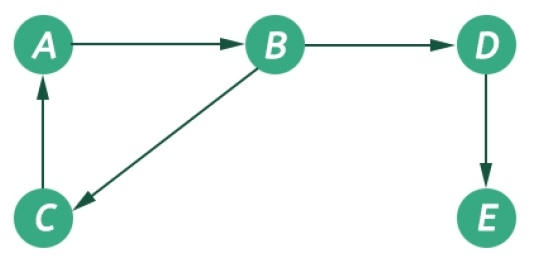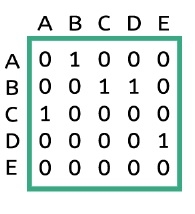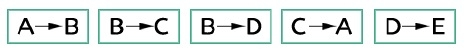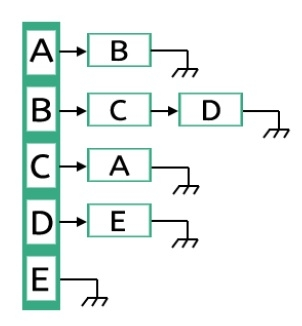# Graph and its representations

The graph is a non-linear data structures. This represents data using nodes, and their relations using edges. A graph G has two sections. The vertices, and edges. Vertices are represented using set V, and Edges are represented as set E. So the graph notation is G(V,E). Let us see one example to get the idea.In this graph, there are five vertices and five edges. The edges are directed. As an example, if we choose the edge connecting vertices B and D, the source vertex is B and destination is D. So we can move B to D but not move from D to B.

The graphs are non-linear, and it has no regular structure. To represent a graph in memory, there are few different styles. These styles are −

• Edge list representation

We can represent a graph using Adjacency matrix. The given matrix is an adjacency matrix. It is a binary, square matrix and from ith row to jth column, if there is an edge, that place is marked as 1. When we will try to represent an undirected graph using adjacency matrix, the matrix will be symmetric.## Edge List RepresentationGraphs can be represented using one dimensional array also. This is called the edge list. In this representation there are five edges are present, for each edge the first element is the source and the second one is the destination. For undirected graph representation the number of elements in the edge list will be doubled.